Geometry
Operations
Place Value
Fractions
100

What is the name of each shape?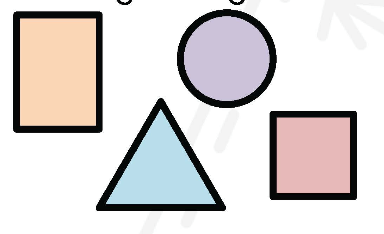Rectangle

Circle

Triangle

Square

100

Find the sum: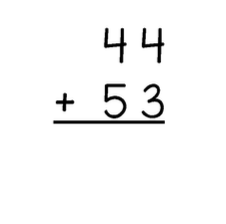The sum is 97

100

Round to the nearest 10.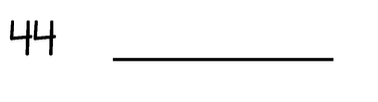40

100

Compare: >,< or =

1/2 ______ 3/4

<

100

How do you find Area?

Area = Length x Width

200

Which shape(s) only have right angles?Rectangle and Square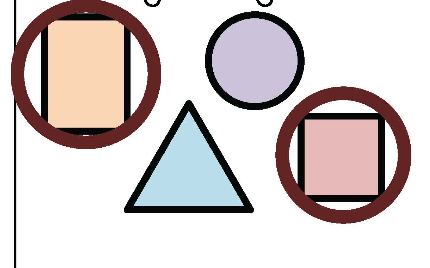200

Find the product:

5 xx 7

The product is 35

200

Round to the nearest 10:

208

210

200

Compare: >,< or =

1/2 _____ 5/10

=

200

How do you find Perimeter?

Perimeter = 2L + 2W OR L + L + W + W

300

What type of lines are these?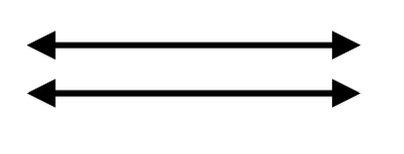Parallel lines (Never cross)

300

Find the quotient:

16 -: 4

The quotient is: 4

300

Round to the nearest 100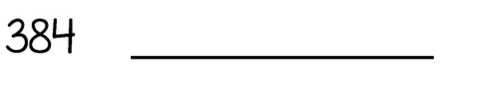400

300

Solve:

4/10 + 5/10

9/10

300

Find the area of the square:* A square has 4 equal sides*

Area = 8 x 8 = 64 squared m

400

What type of lines are these?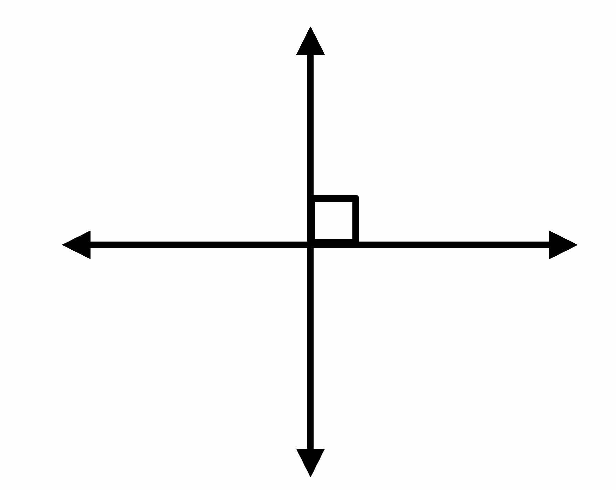Perpendicular lines (cross at a 90 degree angle)

400

Find the sum: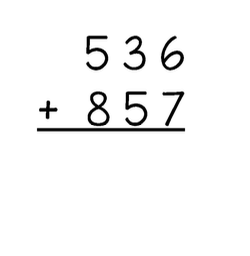The sum is: 1,393

400

Round to the nearest 100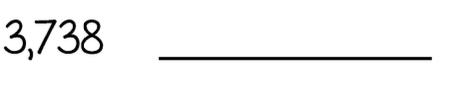3,700

400

Solve :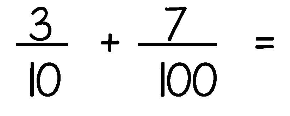37/100

400

Find the perimeter of the rectangle: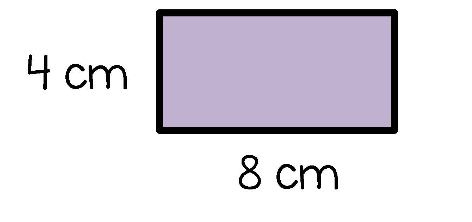Perimeter = 24 cm

500

Name the shape below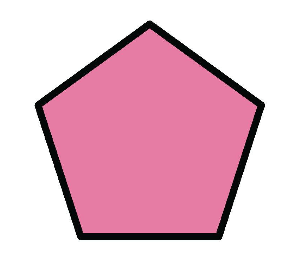Pentagon (it has 5 sides)

500

Find the difference:The difference is:  128

500

Round to the nearest 1,000

35,258

35,000

500

Solve:

99/100 - 2/10

79/100

500

Find the area of the square: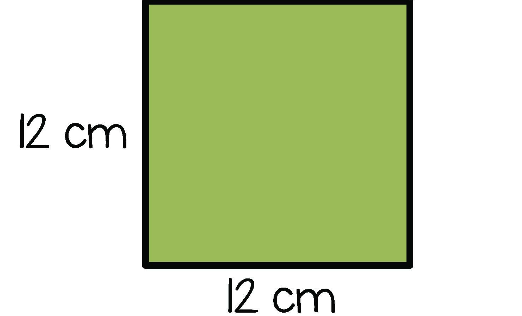Area = 144 squared cm

Click to zoom